# SAT Math Multiple Choice Question 683: Answer and Explanation

### Test Information

Question: 683

8. If the expression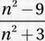is equivalent to the expression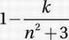for all values of n, what is the value of k?

• A. -12
• B. -6
• C. 6
• D. 12

Explanation:

D

Given equation: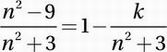Add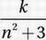: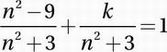Combine the fractions into one: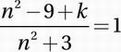Multiply by n2 + 3:

n2 - 9 + k = n2 + 3

Subtract n2 :

-9 + k = 3